Win up to 100% scholarship on Aakash BYJU'S JEE/NEET courses with ABNAT Win up to 100% scholarship on Aakash BYJU'S JEE/NEET courses with ABNAT

# Bond Enthalpy

## Introduction: What Is Bond Enthalpy?

Bond enthalpy, also known as bond energy, is a quantity that offers insight into the strength of a chemical bond and, by extension, its stability. The bond enthalpy of a chemical bond can be defined as the total amount of energy required to break 1 mole of that chemical bond. For example, the bond enthalpy of the oxygen-hydrogen single bond is equal to 463 kJ/mol. This implies that a total of 463 kilojoules of energy is required to break 1 mole of hydrogen-oxygen single bonds.

It is important to note that the breaking of a chemical bond is always an endothermic process (because energy must be supplied to the molecule in order to break the chemical bonds that constitute it). Thus, the enthalpy change associated with the breaking of a chemical bond is always positive (ΔH > 0). On the other hand, the formation of a chemical bond is usually an endothermic process. In such cases, the enthalpy change will have a negative value (ΔH < 0).

To express the strength of a single, specific bond in a molecule, the quantity ‘mean bond enthalpy’ or ‘average bond enthalpy’ can be used. The mean bond energy of a chemical bond in a molecule can be determined by calculating the average value of all the bond dissociation energies of that type of bond in the molecule. Thus, mean bond enthalpy is different from bond dissociation energy (except for diatomic molecules).

It can also be noted that some definitions specifically define bond enthalpy as the amount of energy required to break one mole of a chemical bond at 298K at the gas phase. The higher the value, the stronger the bond and the high energy required to break the bond. An illustration detailing how the chemical bond between atoms A and B is broken when energy equal to the bond enthalpy is supplied to molecule AB is provided below.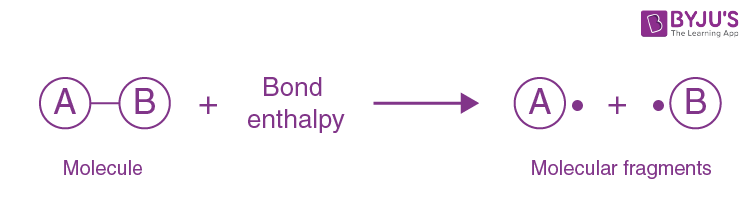For example, the bond dissociation energy required to break 1 mole of gaseous hydrogen chloride molecule to gaseous hydrogen and chlorine atom requires 432kJ, and bond dissociation enthalpy of gaseous HCl is +432kJ per mol.

If a molecule has several bonds, bond enthalpy is calculated for each bond, and the average value is considered. For example, methane (CH4) has four C-H bonds, and the average bond energy is +1652 kJ and +415.5kJ per mole of the bond.

Note: Bond enthalpy can be calculated directly if everything you are working on is in a gaseous state. If it is in the liquid state, it needs extra energy to convert it from the liquid state to the gaseous state

## Average Bond Enthalpies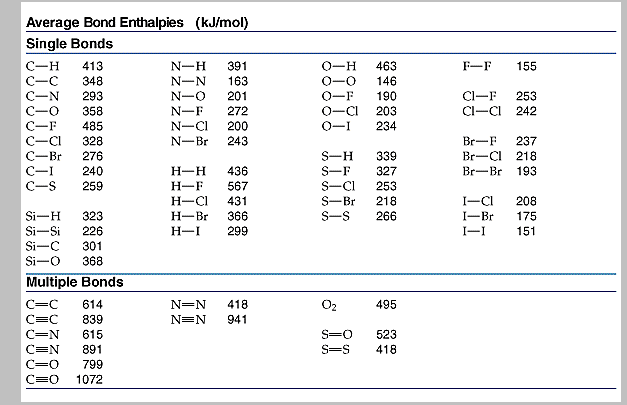Bond enthalpy to estimate the enthalpy of the reaction

Consider an example of hydrogenation of propene which has the following steps.

• Breaking of carbon-carbon double bond and H-H bond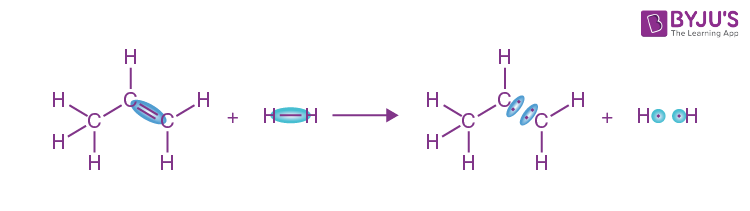Using the reference table bond enthalpy of C double bond C is 610kJ/mol, while the H-H bond is 436kJ/mol,

• Calculate the energy required to break the C-C double bond and H-H bond, and the addition of this gives the total amount of energy required to break the desired bonds in propene and hydrogen gas, that is, 1046kJ/mol(610kJ/mol+ 436 Kj/mol= 1046 kJ/mol).
• The reaction gives the new molecule forming one C-C bond and two C-H bonds.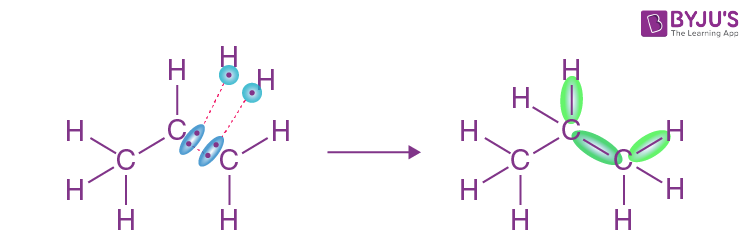In order to understand how much energy is released during the process, calculate the bond enthalpy of the C-C bond and multiply by -1 and the bond enthalpy of the C-H bond by 2; combining the bond enthalpy gives the amount of energy released during the process.

Energy released to make product bond = -346kJ/mol + (2* -413kJ/mol) = -1172kJ/mol

• From the energy of the above step required to break the bond and energy released in the formation of new bonds gives the change in enthalpy during the reaction.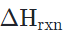= Energy required to break the bond + Energy released in the formation of a new bond

= 1046 kJ/mol+ (-1172/ kJ/mol)

= -126 kJ/mol.

## Endothermic and Exothermic Process

During the reaction, energy is absorbed to break the bond, which is called the endothermic process and energy is released to form a new bond is called the exothermic process. The two processes can be explained in the energy diagram as follows:

• In exothermic reactions, reactants are in higher energy than products, and the energy difference between them is called the change in enthalpy of the reaction (∆H) and is always negative.
• In endothermic reactions, products are in higher energy than reactants, and the energy difference((∆H) between them is always positive.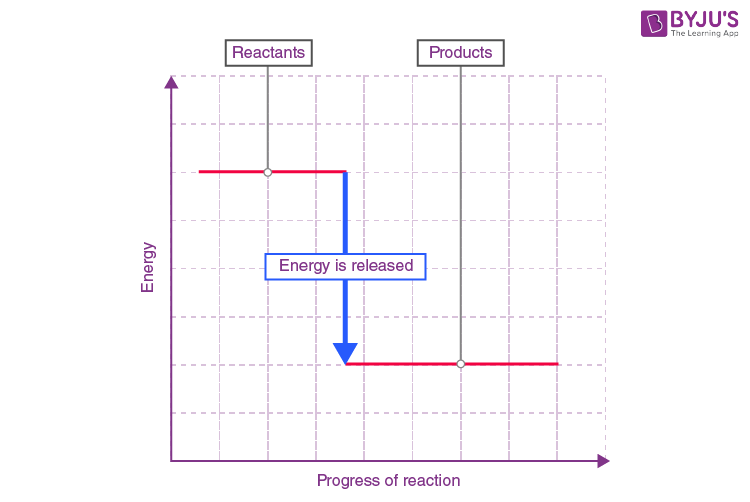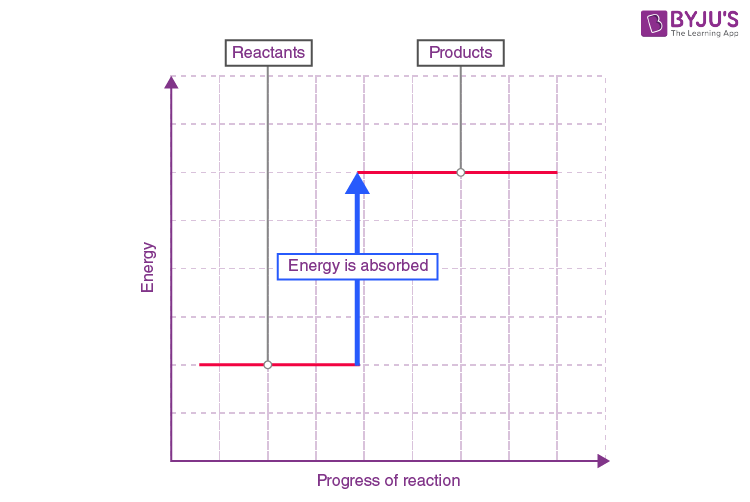### Summary

• Bond enthalpy describes how much energy is required to break or form the bond.
• Combined bond enthalpy for all broken and formed bonds during the process gives the idea of a total change in the energy of the system, which is called the change in enthalpy.
• Depending on whether the change in enthalpy is positive or negative, we can determine whether the reaction is endothermic or exothermic.

## Solved Examples

Calculate the change in enthalpy of the following reactions

1.

$$\begin{array}{l}CH_{4}+Cl_{2}\rightarrow CHCl_{3}+HCl\end{array}$$
(Exothermic reactions)

Solution:

Energy is required to break or make one mole of a particular bond in kJ/mol

C-H = 412kJ/mol, Cl-Cl = 242kJ/mol, C-Cl = 331kJ/mol, H-Cl = 432kJ/mol

Step 1:

The total energy required to break one C-H and Cl-Cl bond is

412kJ/mol +241kJ/mol = 654kJ/mol.

Step 2:

The energy released during the formation of new C-Cl and H-Cl bond is

331kJ/mol + 432kJ/mol = 763kJ/mol.

Step 3:

Energy change = Energy absorbed while breaking the bond – Energy released while forming the bond

Energy change = 654kJ/mol – 763kJ/mol

= -109kJ/mol.

2. HBr(g) ==> H2(g) + Br2(g) ( Endothermic reactions)

Solution:

Energy is required to break or make one mole of a particular bond in kJ/mol

H–Br = 366kJ/mol, H–H = 436kJ/mol, Br–Br = 193kJ/mol

Step 1:

Energy need to break the bonds = 366kJ/mol +366kJ/mol = 732kJ/mol

Step 2:

Energy released on bond formation = 436kJ/mol + 193kJ/mol = 629kJ/mol

Step 3:

Change in enthalpy = 366kJ/mol – 629kJ/mol = +103kJ/mol

3. methane + oxygen ==> carbon dioxide + water(Exothermic process)

CH4(g) + 2O2(g) ==> CO2(g) + 2H2O(g)

Bond energies in kJ/mol

C-H single bond = 412 kJ/mol, O=O double bond = 496 kJ/mol, C=O double bond = 803kJ/mol, H-O single bond = 463 kJ/mol

Solution

Step 1:

Energy required to break the bonds = 4*(C-H) + 2*(1* O=O)

= (4*412) + (2*496)

= 1648 + 992

= 2640kJ/mol.

Step 2:

Energy released while forming bonds = (2*C=O) + 2*(2*O-H)

= (2*208) + 2*(2*463)

= 1606 + 1852

= 3458 kJ/mol.

Step 3:

Change in enthalpy = Step 1 – Step 2

= 2640kJ/mol – 3458 kJ/mol

= -818 kJ/mol.Test your knowledge on Bond Enthalpy